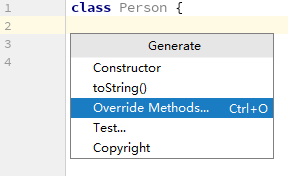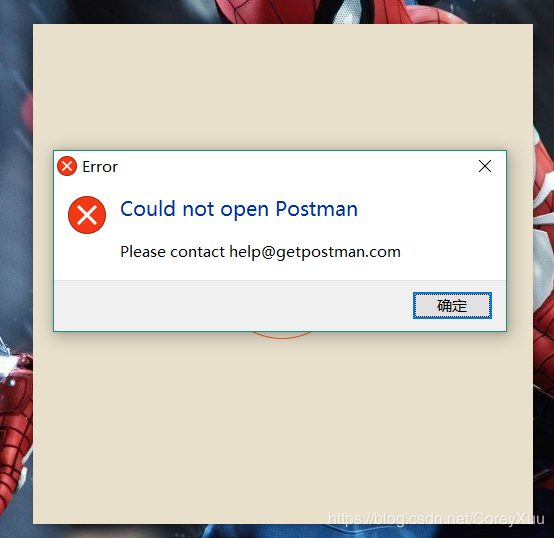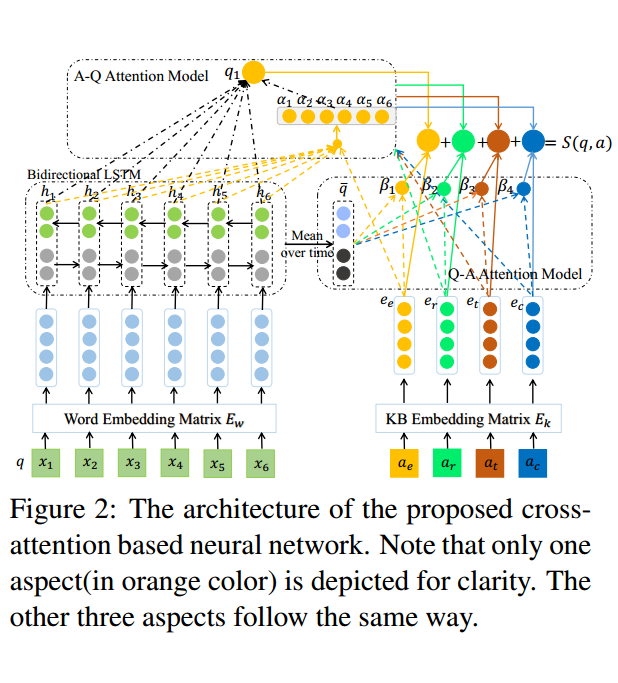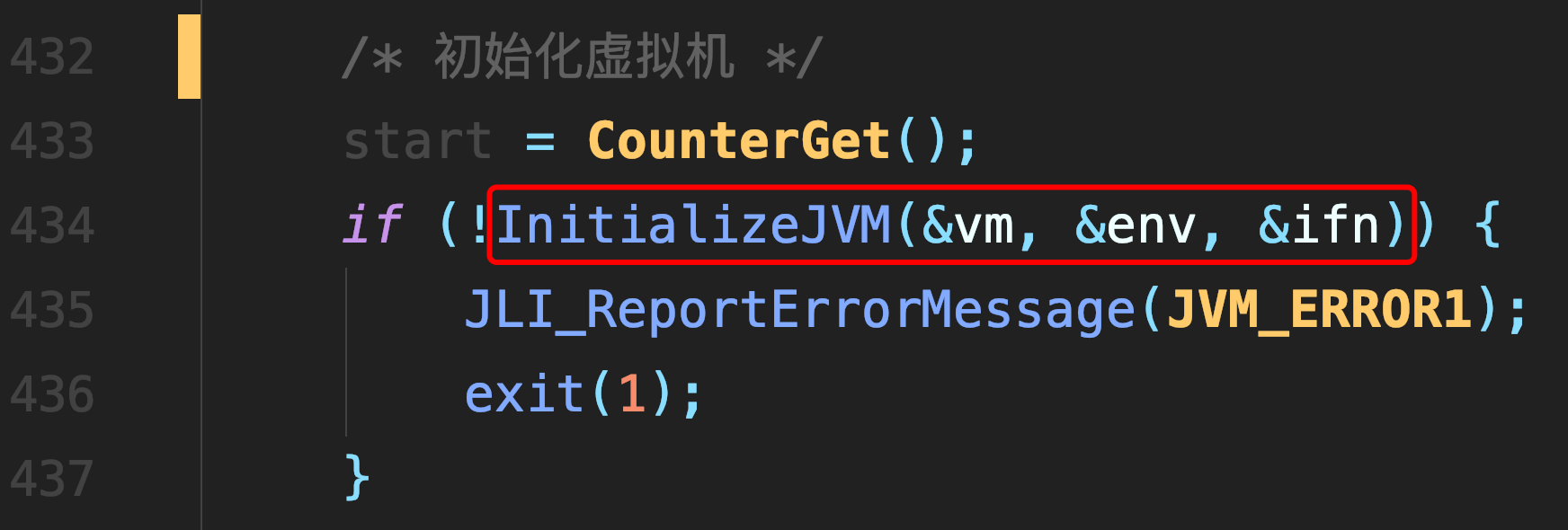# 1034. Head of a Gang (30)

100 ms

65536 kB

16000 B

Standard

CHEN, Yue

One way that the police finds the head of a gang is to check people's phone calls. If there is a phone call between A and B, we say that A and B is related. The weight of a relation is defined to be the total time length of all the phone calls made between the two persons. A "Gang" is a cluster of more than 2 persons who are related to each other with total relation weight being greater than a given threshold K. In each gang, the one with maximum total weight is the head. Now given a list of phone calls, you are supposed to find the gangs and the heads.

Input Specification:

Each input file contains one test case. For each case, the first line contains two positive numbers N and K (both less than or equal to 1000), the number of phone calls and the weight threthold, respectively. Then N lines follow, each in the following format:

Name1 Name2 Time

where Name1 and Name2 are the names of people at the two ends of the call, and Time is the length of the call. A name is a string of three capital letters chosen from A-Z. A time length is a positive integer which is no more than 1000 minutes.

Output Specification:

For each test case, first print in a line the total number of gangs. Then for each gang, print in a line the name of the head and the total number of the members. It is guaranteed that the head is unique for each gang. The output must be sorted according to the alphabetical order of the names of the heads.

Sample Input 1:
8 59
AAA BBB 10
BBB AAA 20
AAA CCC 40
DDD EEE 5
EEE DDD 70
FFF GGG 30
GGG HHH 20
HHH FFF 10

Sample Output 1:
2
AAA 3
GGG 3

Sample Input 2:
8 70
AAA BBB 10
BBB AAA 20
AAA CCC 40
DDD EEE 5
EEE DDD 70
FFF GGG 30
GGG HHH 20
HHH FFF 10

Sample Output 2:
0

``````//说明，由于题目时间的限制，不能使用map容器，但可以自己构造
#include<iostream>
#include<cstdio>
#include<algorithm>
#include<string.h>
using namespace std;
char M; //建立一个自己定义的map

int F; //父节点
int totalTime; //每个人总共通话多久
int sequeue; //通话人的序列

int UFind(int x) //find的功能
{
if(x != F[x])  x = UFind(F[x]);
return x;
}

int Union(int x, int y)
{
if(UFind(x) == UFind(y)) return 0;
x= UFind(x); y = UFind(y);
if(totalTime[x] > totalTime[y])
{
F[y] = x;
}
else F[x] = y;
return 0;
}

int main()
{
//freopen("E:/in.txt", "r", stdin);
int n, limit;
scanf("%d%d", &n, &limit);

char tempS1, tempS2;
int tempN1, tempN2, times;
int j = 0;
for(int i = 0; i < n; i++)
{
scanf("%s%s%d", tempS1, tempS2, ×);
tempN1 = (tempS1 - 'A')*26*26 + (tempS1 - 'A')*26 + tempS1 - 'A';
tempN2 = (tempS2 - 'A')*26*26 + (tempS2 - 'A')*26 + tempS2 - 'A';
strcpy(M[tempN1], tempS1);  strcpy(M[tempN2], tempS2);
F[tempN1] = tempN1; F[tempN2] = tempN2;
totalTime[tempN1] += times;  totalTime[tempN2] += times;
sequeue[j++] = tempN1; sequeue[j++] = tempN2;
}

for(int i = 0; i < j; i++)
{
Union(sequeue[i], sequeue[i+1]); //组成一个群
i++;
}

bool flag;
int len; int together;
memset(flag, false, sizeof(flag));
memset(together, 0, sizeof(together));
memset(len, 0, sizeof(len));

int father;
for(int i = 0; i < j; i++)
{
if(!flag[sequeue[i]])
{
father = UFind(sequeue[i]);
len[father]++;
together[father] += totalTime[sequeue[i]];
flag[sequeue[i]] = true;
}
}

memset(flag, false, sizeof(flag));
int result; int w = 0;
for(int i = 0; i < j; i++)
{
father = UFind(sequeue[i]);
if(!flag[father])
{
if(len[father] > 2 && together[father] > 2 * limit)
{
result[w++] = father;
flag[father] = true;
}
}
}
if(w == 0)
{
printf("0\n");
return 0;
}
sort(result, result+w);

printf("%d\n", w);
for(int i = 0; i < w; i++)
{
printf("%s %d\n", M[result[i]], len[result[i]]);
}
return 0;
}
``````

##### 热门推荐
•浏览(655)
•浏览(565)
•浏览(548)
•浏览(488)
•浏览(434)
•浏览(424)
•浏览(381)
•浏览(367)
•浏览(362)
•浏览(358)